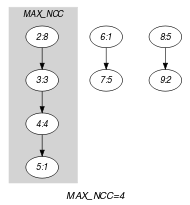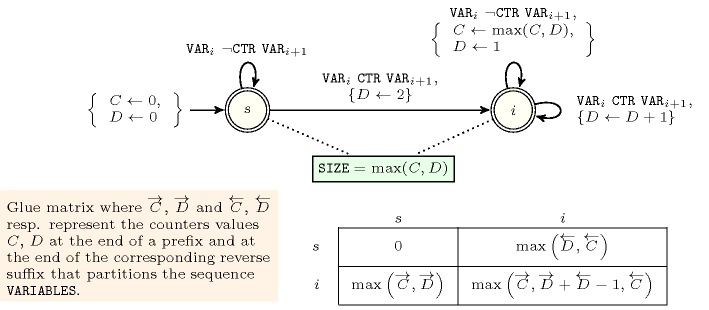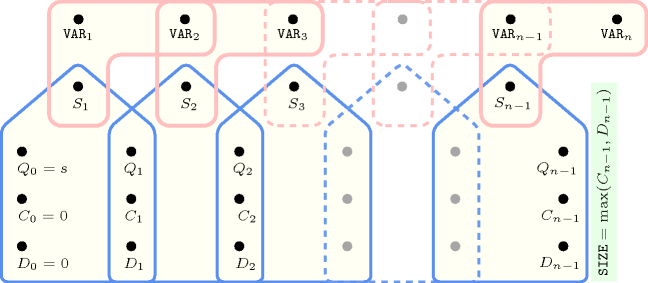## 5.235. longest_change

Origin

Derived from $\mathrm{𝚌𝚑𝚊𝚗𝚐𝚎}$.

Constraint

$\mathrm{𝚕𝚘𝚗𝚐𝚎𝚜𝚝}_\mathrm{𝚌𝚑𝚊𝚗𝚐𝚎}\left(\mathrm{𝚂𝙸𝚉𝙴},\mathrm{𝚅𝙰𝚁𝙸𝙰𝙱𝙻𝙴𝚂},\mathrm{𝙲𝚃𝚁}\right)$

Arguments
 $\mathrm{𝚂𝙸𝚉𝙴}$ $\mathrm{𝚍𝚟𝚊𝚛}$ $\mathrm{𝚅𝙰𝚁𝙸𝙰𝙱𝙻𝙴𝚂}$ $\mathrm{𝚌𝚘𝚕𝚕𝚎𝚌𝚝𝚒𝚘𝚗}\left(\mathrm{𝚟𝚊𝚛}-\mathrm{𝚍𝚟𝚊𝚛}\right)$ $\mathrm{𝙲𝚃𝚁}$ $\mathrm{𝚊𝚝𝚘𝚖}$
Restrictions
 $\mathrm{𝚂𝙸𝚉𝙴}\ge 0$ $\mathrm{𝚂𝙸𝚉𝙴}\le |\mathrm{𝚅𝙰𝚁𝙸𝙰𝙱𝙻𝙴𝚂}|$ $\mathrm{𝚛𝚎𝚚𝚞𝚒𝚛𝚎𝚍}$$\left(\mathrm{𝚅𝙰𝚁𝙸𝙰𝙱𝙻𝙴𝚂},\mathrm{𝚟𝚊𝚛}\right)$ $\mathrm{𝙲𝚃𝚁}\in \left[=,\ne ,<,\ge ,>,\le \right]$
Purpose

$\mathrm{𝚂𝙸𝚉𝙴}$ is the maximum number of consecutive variables of the collection $\mathrm{𝚅𝙰𝚁𝙸𝙰𝙱𝙻𝙴𝚂}$ for which constraint $\mathrm{𝙲𝚃𝚁}$ holds in an uninterrupted way (0 if the constraint $\mathrm{𝙲𝚃𝚁}$ does not hold at all). We count a change when $X\mathrm{𝙲𝚃𝚁}Y$ holds; $X$ and $Y$ are two consecutive variables of the collection $\mathrm{𝚅𝙰𝚁𝙸𝙰𝙱𝙻𝙴𝚂}$.

Example
$\left(4,〈8,8,3,4,1,1,5,5,2〉,\ne \right)$

The $\mathrm{𝚕𝚘𝚗𝚐𝚎𝚜𝚝}_\mathrm{𝚌𝚑𝚊𝚗𝚐𝚎}$ constraint holds since its first argument $\mathrm{𝚂𝙸𝚉𝙴}=4$ is fixed to the length of the longest subsequence of consecutive values of the collection $〈8,8,3,4,1,1,5,5,2〉$ such that two consecutive values are distinct (i.e., subsequence $8341$).

Typical
 $|\mathrm{𝚅𝙰𝚁𝙸𝙰𝙱𝙻𝙴𝚂}|>2$ $\mathrm{𝚛𝚊𝚗𝚐𝚎}$$\left(\mathrm{𝚅𝙰𝚁𝙸𝙰𝙱𝙻𝙴𝚂}.\mathrm{𝚟𝚊𝚛}\right)>1$ $\mathrm{𝙲𝚃𝚁}\in \left[\ne \right]$
Symmetry

One and the same constant can be added to the $\mathrm{𝚟𝚊𝚛}$ attribute of all items of $\mathrm{𝚅𝙰𝚁𝙸𝙰𝙱𝙻𝙴𝚂}$.

Arg. properties

Functional dependency: $\mathrm{𝚂𝙸𝚉𝙴}$ determined by $\mathrm{𝚅𝙰𝚁𝙸𝙰𝙱𝙻𝙴𝚂}$ and $\mathrm{𝙲𝚃𝚁}$.

Keywords
Arc input(s)

$\mathrm{𝚅𝙰𝚁𝙸𝙰𝙱𝙻𝙴𝚂}$

Arc generator
$\mathrm{𝑃𝐴𝑇𝐻}$$↦\mathrm{𝚌𝚘𝚕𝚕𝚎𝚌𝚝𝚒𝚘𝚗}\left(\mathrm{𝚟𝚊𝚛𝚒𝚊𝚋𝚕𝚎𝚜}\mathtt{1},\mathrm{𝚟𝚊𝚛𝚒𝚊𝚋𝚕𝚎𝚜}\mathtt{2}\right)$

Arc arity
Arc constraint(s)
$\mathrm{𝚟𝚊𝚛𝚒𝚊𝚋𝚕𝚎𝚜}\mathtt{1}.\mathrm{𝚟𝚊𝚛}\mathrm{𝙲𝚃𝚁}\mathrm{𝚟𝚊𝚛𝚒𝚊𝚋𝚕𝚎𝚜}\mathtt{2}.\mathrm{𝚟𝚊𝚛}$
Graph property(ies)
$\mathrm{𝐌𝐀𝐗}_\mathrm{𝐍𝐂𝐂}$$=\mathrm{𝚂𝙸𝚉𝙴}$

Graph model

In order to specify the $\mathrm{𝚕𝚘𝚗𝚐𝚎𝚜𝚝}_\mathrm{𝚌𝚑𝚊𝚗𝚐𝚎}$ constraint, we use $\mathrm{𝐌𝐀𝐗}_\mathrm{𝐍𝐂𝐂}$, which is the number of vertices of the largest connected component. Since the initial graph corresponds to a path, this will be the length of the longest path in the final graph.

Parts (A) and (B) of Figure 5.235.1 respectively show the initial and final graph associated with the Example slot. Since we use the $\mathrm{𝐌𝐀𝐗}_\mathrm{𝐍𝐂𝐂}$ graph property we show the largest connected component of the final graph. It corresponds to the longest period of uninterrupted changes: sequence $8,3,4,1$ that involves 4 consecutive variables.

##### Figure 5.235.1. Initial and final graph of the $\mathrm{𝚕𝚘𝚗𝚐𝚎𝚜𝚝}_\mathrm{𝚌𝚑𝚊𝚗𝚐𝚎}$ constraint(a) (b)
Automaton

Figure 5.235.2 depicts the automaton associated with the $\mathrm{𝚕𝚘𝚗𝚐𝚎𝚜𝚝}_\mathrm{𝚌𝚑𝚊𝚗𝚐𝚎}$ constraint. To each pair of consecutive variables $\left({\mathrm{𝚅𝙰𝚁}}_{i},{\mathrm{𝚅𝙰𝚁}}_{i+1}\right)$ of the collection $\mathrm{𝚅𝙰𝚁𝙸𝙰𝙱𝙻𝙴𝚂}$ corresponds a 0-1 signature variable ${S}_{i}$. The following signature constraint links ${\mathrm{𝚅𝙰𝚁}}_{i}$, ${\mathrm{𝚅𝙰𝚁}}_{i+1}$ and ${S}_{i}$: ${\mathrm{𝚅𝙰𝚁}}_{i}\mathrm{𝙲𝚃𝚁}{\mathrm{𝚅𝙰𝚁}}_{i+1}⇔{S}_{i}$.

##### Figure 5.235.2. Automaton of the $\mathrm{𝚕𝚘𝚗𝚐𝚎𝚜𝚝}_\mathrm{𝚌𝚑𝚊𝚗𝚐𝚎}$ constraint and its glue matrix##### Figure 5.235.3. Hypergraph of the reformulation corresponding to the automaton of the $\mathrm{𝚕𝚘𝚗𝚐𝚎𝚜𝚝}_\mathrm{𝚌𝚑𝚊𝚗𝚐𝚎}$ constraint